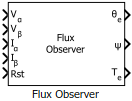Main Content

# Flux Observer

Compute electrical position, magnetic flux, and electrical torque of rotor

• Library:
• Motor Control Blockset / Sensorless Estimators

•## Description

The Flux Observer block computes the electrical position, magnetic flux, and electrical torque of a PMSM or an induction motor by using the per unit voltage and current values along the α- and β-axes in the stationary αβ reference frame.

### Equations

These equations describe how the block computes the electrical position, magnetic flux, and electrical torque for a PMSM.

`${T}_{\text{e}}=\frac{3}{2}P\left({\psi }_{\alpha }{I}_{\beta }-{\psi }_{\beta }{I}_{\alpha }\right)$`

These equations describe how the block computes the rotor electrical position, rotor magnetic flux, and electrical torque for an induction motor.

`$\sigma =1-\frac{{L}_{m}^{2}}{{L}_{r}\cdot {L}_{s}}$`

`${T}_{\text{e}}=\frac{3}{2}\cdot P\cdot \frac{{L}_{m}}{{L}_{r}}\left({\psi }_{\alpha }{I}_{\beta }-{\psi }_{\beta }{I}_{\alpha }\right)$`

where:

• ${V}_{\alpha }$ and ${V}_{\beta }$ are the α-axis and β-axis voltages (Volts).

• ${I}_{\alpha }$ and ${I}_{\beta }$ are the α-axis and β-axis current (Amperes).

• $R$ is the stator resistance of the motor (Ohms).

• ${L}_{s}$ is the stator inductance of the motor (Henry).

• ${L}_{r}$ is the rotor inductance of the motor (Henry).

• ${L}_{m}$ is the magnetizing inductance of the motor (Henry).

• $\sigma$ is the total leakage factor of the induction motor.

• $P$ is the number of motor pole pairs.

• $\psi$ is the rotor magnetic flux (Weber).

• ${\psi }_{\alpha }$ and ${\psi }_{\beta }$ are the rotor magnetic fluxes along the α- and β-axes (Weber).

• ${T}_{e}$ is the electrical torque of the rotor (Nm).

• ${\theta }_{e}$ is the electrical position of the rotor (Radians).

## Ports

### Input

expand all

Voltage component along the α-axis in the stationary αβ reference frame.

Data Types: `single` | `double` | `fixed point`

Voltage component along the β-axis in the stationary αβ reference frame.

Data Types: `single` | `double` | `fixed point`

Current along the α-axis in the stationary αβ reference frame.

Data Types: `single` | `double` | `fixed point`

Current along the β-axis in the stationary αβ reference frame.

Data Types: `single` | `double` | `fixed point`

The pulse (true value) that resets the block algorithm.

Data Types: `single` | `double` | `fixed point`

### Output

expand all

The electrical position of the rotor as estimated by the block.

#### Dependencies

To enable this port, set Block output to `Position`.

Data Types: `single` | `double` | `fixed point`

The magnetic flux of the rotor as estimated by the block.

#### Dependencies

To enable this port, set Block output to `Flux`.

Data Types: `single` | `double` | `fixed point`

The electrical torque of the rotor as estimated by the block.

#### Dependencies

To enable this port, set Block output to `Torque`.

Data Types: `single` | `double` | `fixed point`

## Parameters

expand all

### Motor parameters

Select the type of motor that the block supports.

Select the unit of the α and β-axes voltage and current input values.

Select one or more quantities that the block should compute and display in the block output.

Note

You must select at least one value. The block displays an error message if you click or without selecting any value.

Number of pole pairs available in the motor.

#### Dependencies

To enable this parameter, set Block output to `Torque`.

Stator phase winding resistance of the motor in ohms.

Stator winding inductance of the motor along d-axis in Henry.

#### Dependencies

To enable this parameter, set Motor selection to `PMSM`.

Leakage inductance of the induction motor stator winding in Henry.

#### Dependencies

To enable this parameter, set Motor selection to `ACIM`.

Leakage inductance of the induction motor rotor winding in Henry.

#### Dependencies

To enable this parameter, set Motor selection to `ACIM`.

Magnetizing inductance of the induction motor in Henry.

#### Dependencies

To enable this parameter, set Motor selection to `ACIM`.

Cutoff frequency of the internal high-pass filter (that filters noise) in Hertz.

The Flux Observer block uses an internal first order IIR high-pass filter. You should set the Cutoff frequency (Hz) for this filter to a value that is lower than the lowest frequency corresponding to the minimum speed of the motor. For example, you can enter a value that is one-tenth of the lowest electrical frequency of the stator voltages and the currents. However, you can adjust this value to determine a more accurate cutoff frequency that generates the desired block output.

The fixed time interval in seconds between two consecutive instances of block execution.

### Datatypes

Unit of the electrical position output.

#### Dependencies

To enable this parameter, set Block output to `Position`.

Data type of the electrical position output.

#### Dependencies

To enable this parameter, set Block output to `Position`.

Unit of the magnetic flux output.

#### Dependencies

To enable this parameter, set Block output to `Flux`.

Data type of the magnetic flux output.

#### Dependencies

To enable this parameter, set Block output to `Flux`.

Unit of the electrical torque output.

#### Dependencies

To enable this parameter, set Block output to `Torque`.

Data type of the electrical torque output.

#### Dependencies

To enable this parameter, set Block output to `Torque`.

 O. Sandre-Hernandez, J. J. Rangel-Magdaleno and R. Morales-Caporal, "Simulink-HDL cosimulation of direct torque control of a PM synchronous machine based FPGA," 2014 11th International Conference on Electrical Engineering, Computing Science and Automatic Control (CCE), Campeche, 2014, pp. 1-6. (doi: 10.1109/ICEEE.2014.6978298)

 Y. Inoue, S. Morimoto and M. Sanada, "Control method suitable for direct torque control based motor drive system satisfying voltage and current limitations," The 2010 International Power Electronics Conference - ECCE ASIA -, Sapporo, 2010, pp. 3000-3006. (doi: 10.1109/IPEC.2010.5543698)

Get ebook